Скачать презентацию Exploratory Data Analysis Hal Varian 20 March 2006

793429215245610efc2bc2191be0104a.ppt

• Количество слайдов: 33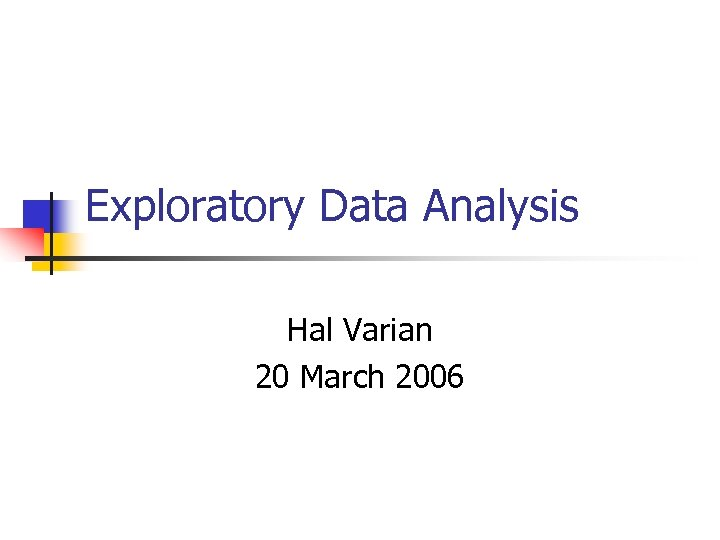Exploratory Data Analysis Hal Varian 20 March 2006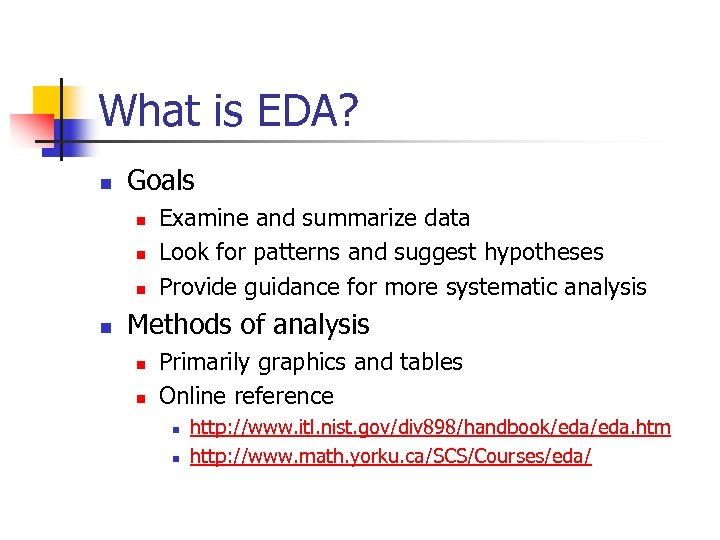What is EDA? n Goals n n Examine and summarize data Look for patterns and suggest hypotheses Provide guidance for more systematic analysis Methods of analysis n n Primarily graphics and tables Online reference n n http: //www. itl. nist. gov/div 898/handbook/eda. htm http: //www. math. yorku. ca/SCS/Courses/eda/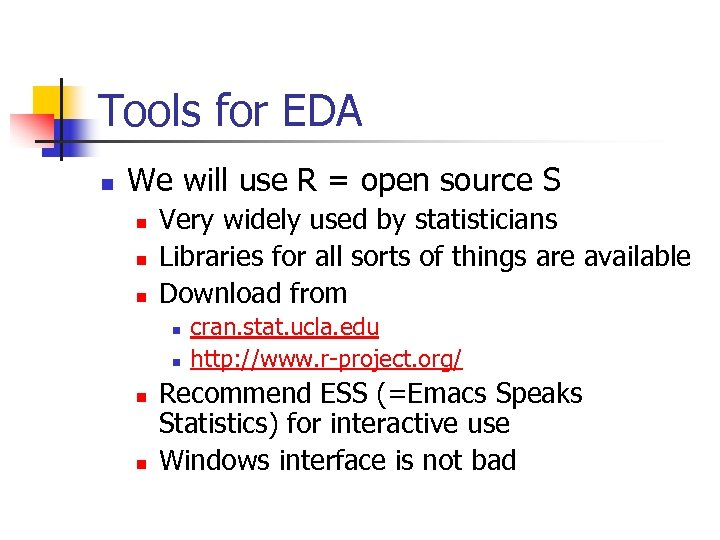Tools for EDA n We will use R = open source S n n n Very widely used by statisticians Libraries for all sorts of things are available Download from n n cran. stat. ucla. edu http: //www. r-project. org/ Recommend ESS (=Emacs Speaks Statistics) for interactive use Windows interface is not bad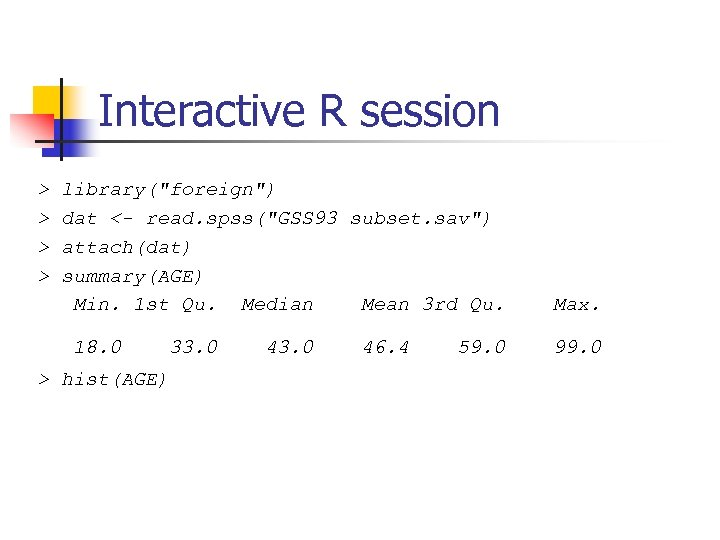Interactive R session > > library("foreign") dat <- read. spss("GSS 93 subset. sav") attach(dat) summary(AGE) Min. 1 st Qu. Median Mean 3 rd Qu. 18. 0 33. 0 > hist(AGE) 43. 0 46. 4 59. 0 Max. 99. 0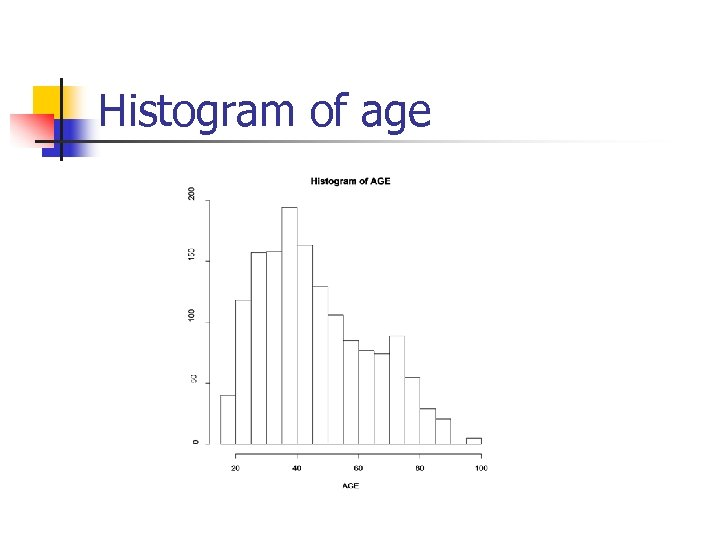Histogram of age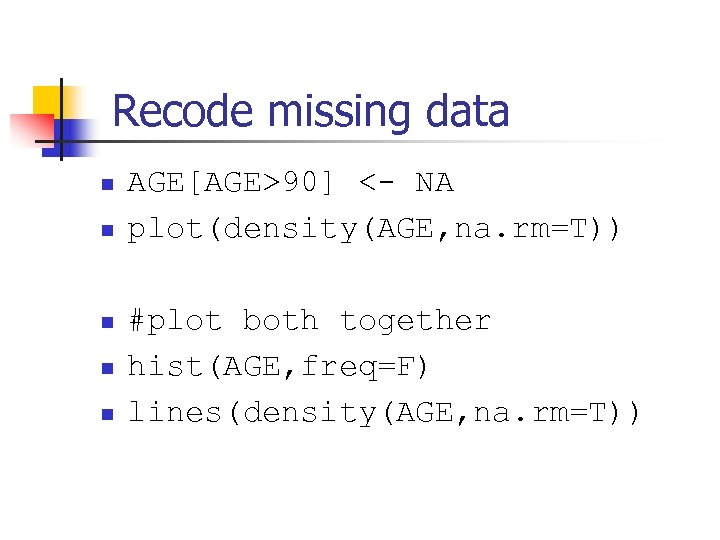Recode missing data n n n AGE[AGE>90] <- NA plot(density(AGE, na. rm=T)) #plot both together hist(AGE, freq=F) lines(density(AGE, na. rm=T))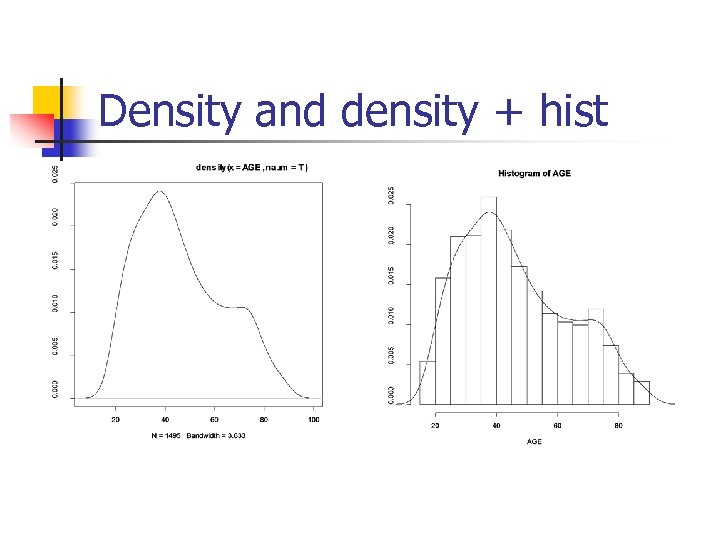Density and density + hist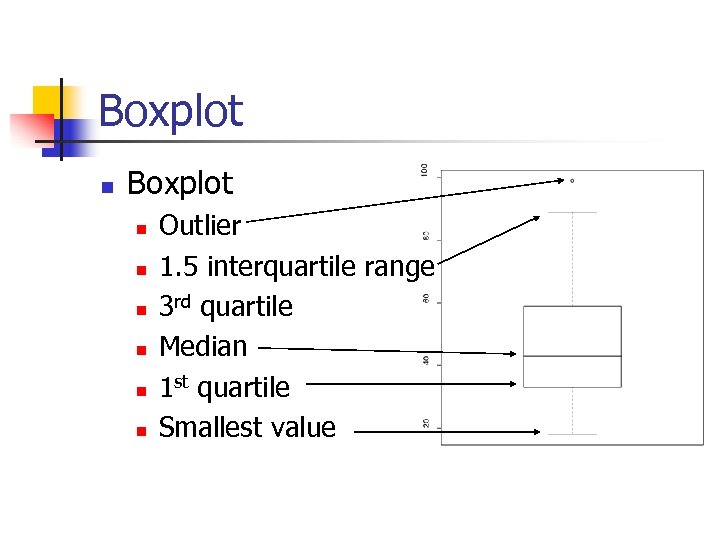Boxplot n n n n Outlier 1. 5 interquartile range 3 rd quartile Median 1 st quartile Smallest value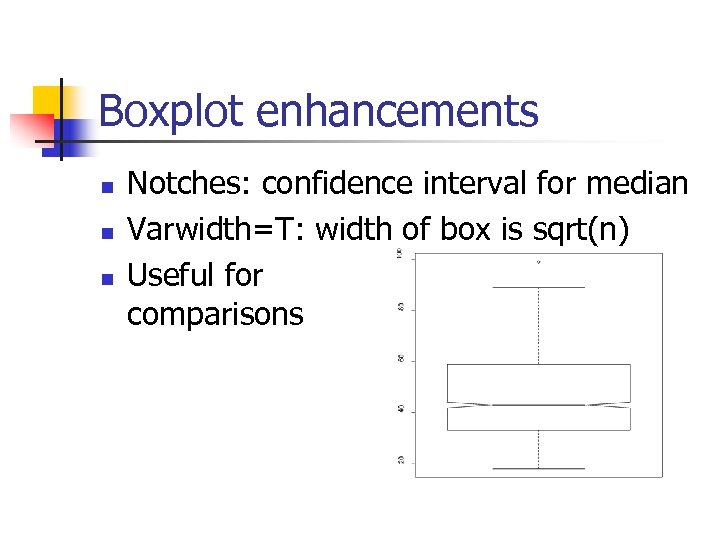Boxplot enhancements n n n Notches: confidence interval for median Varwidth=T: width of box is sqrt(n) Useful for comparisons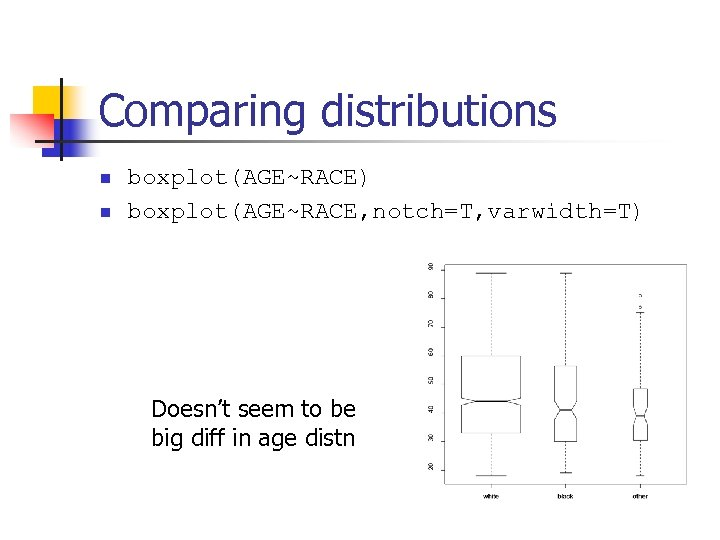Comparing distributions n n boxplot(AGE~RACE) boxplot(AGE~RACE, notch=T, varwidth=T) Doesn’t seem to be big diff in age distn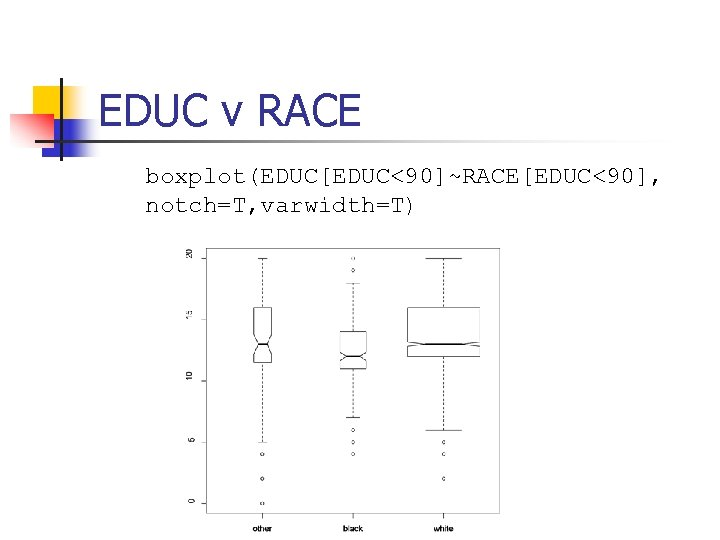EDUC v RACE boxplot(EDUC[EDUC<90]~RACE[EDUC<90], notch=T, varwidth=T)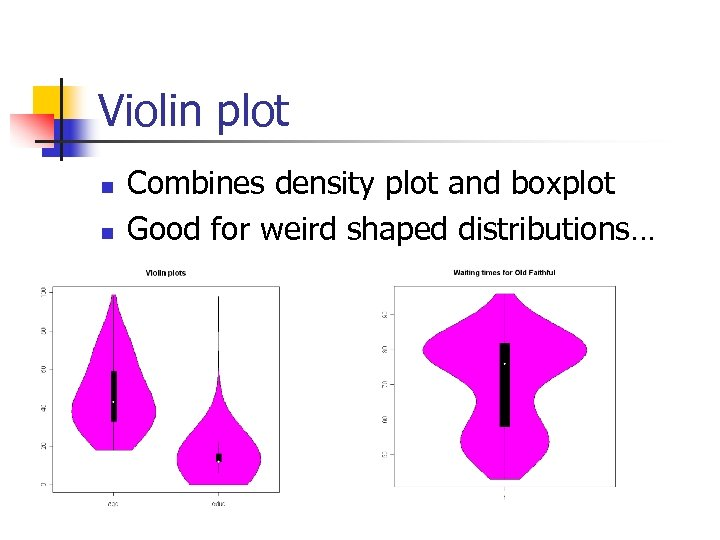Violin plot n n Combines density plot and boxplot Good for weird shaped distributions…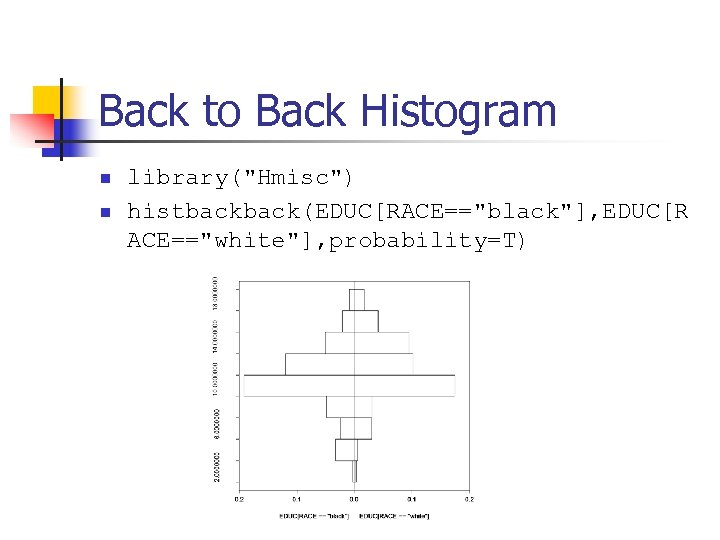Back to Back Histogram n n library("Hmisc") histback(EDUC[RACE=="black"], EDUC[R ACE=="white"], probability=T)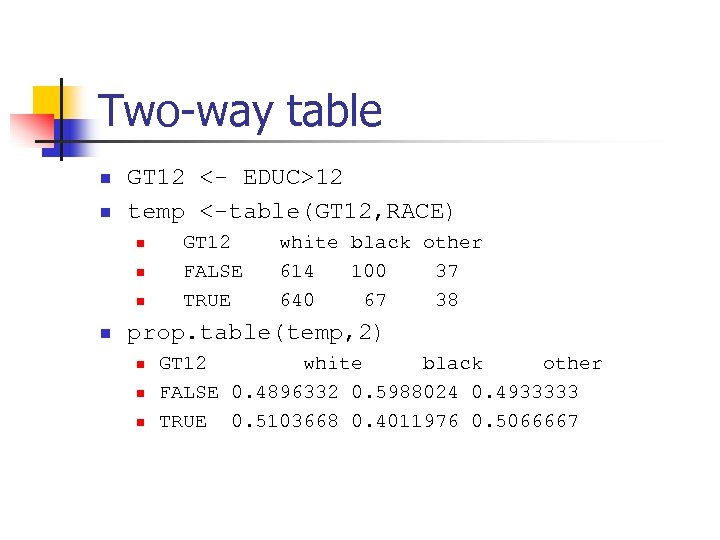Two-way table n n GT 12 <- EDUC>12 temp <-table(GT 12, RACE) n n GT 12 FALSE TRUE white black other 614 100 37 640 67 38 prop. table(temp, 2) n n n GT 12 white black other FALSE 0. 4896332 0. 5988024 0. 4933333 TRUE 0. 5103668 0. 4011976 0. 5066667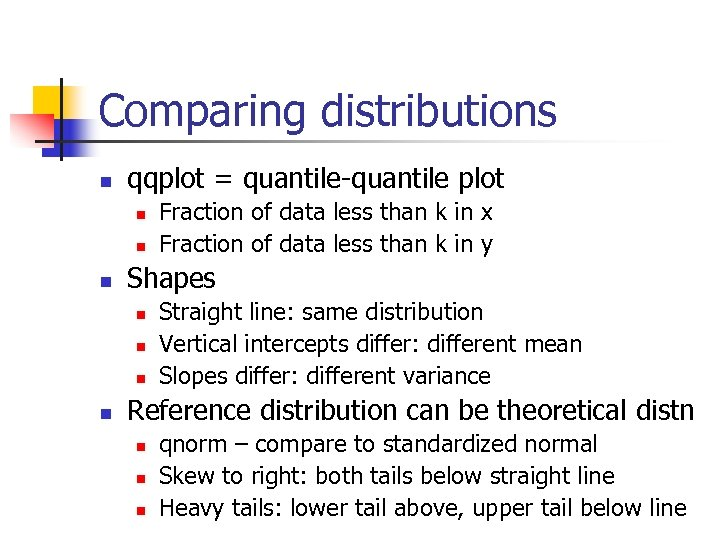Comparing distributions n qqplot = quantile-quantile plot n n n Shapes n n Fraction of data less than k in x Fraction of data less than k in y Straight line: same distribution Vertical intercepts differ: different mean Slopes differ: different variance Reference distribution can be theoretical distn n qnorm – compare to standardized normal Skew to right: both tails below straight line Heavy tails: lower tail above, upper tail below line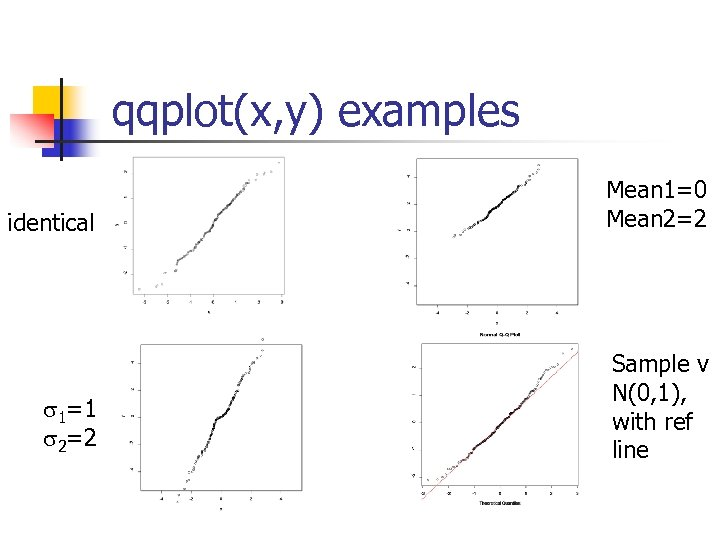qqplot(x, y) examples identical Mean 1=0 Mean 2=2 s 1=1 s 2=2 Sample v N(0, 1), with ref line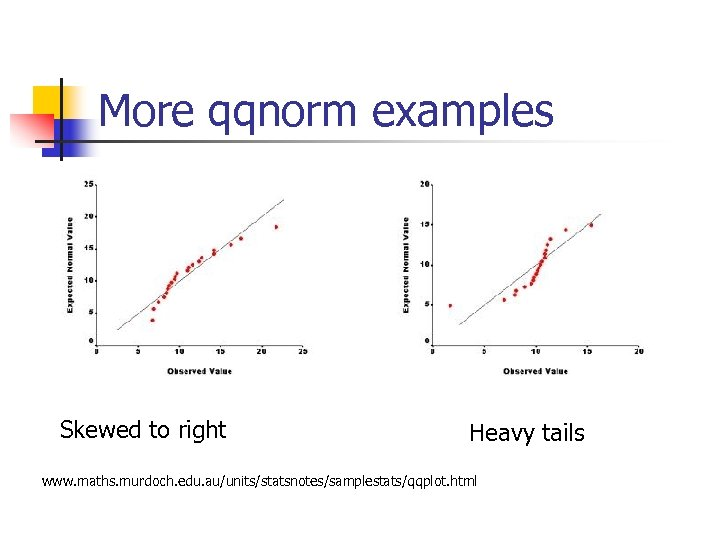More qqnorm examples Skewed to right Heavy tails www. maths. murdoch. edu. au/units/statsnotes/samplestats/qqplot. html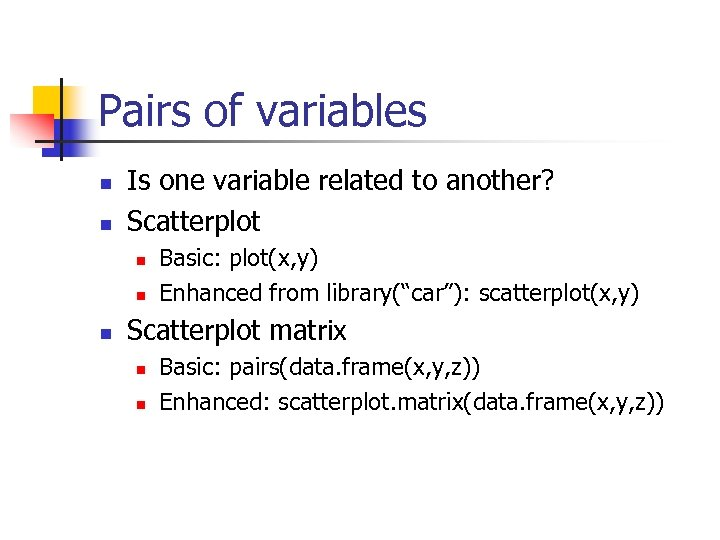Pairs of variables n n Is one variable related to another? Scatterplot n n n Basic: plot(x, y) Enhanced from library(“car”): scatterplot(x, y) Scatterplot matrix n n Basic: pairs(data. frame(x, y, z)) Enhanced: scatterplot. matrix(data. frame(x, y, z))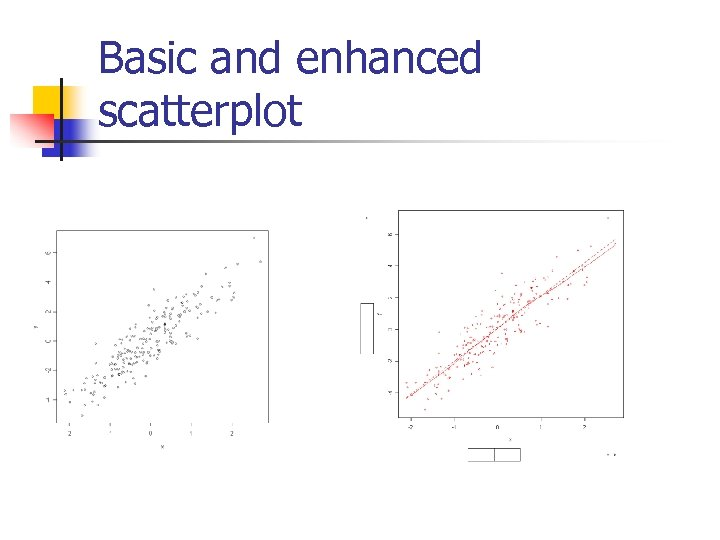Basic and enhanced scatterplot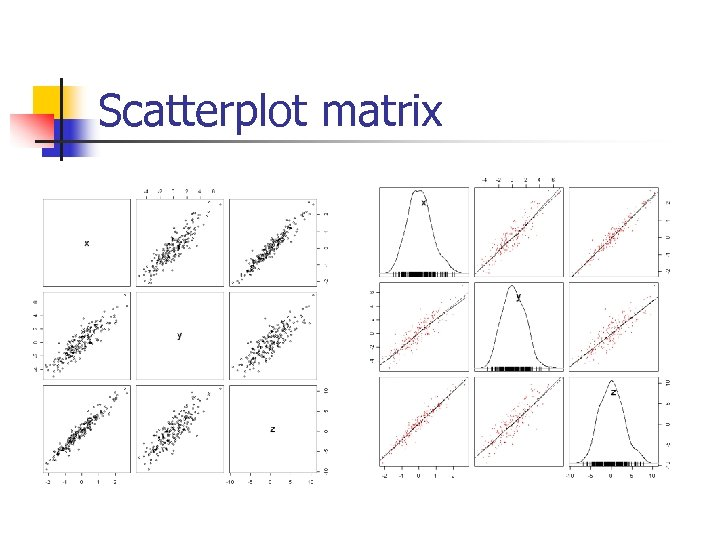Scatterplot matrix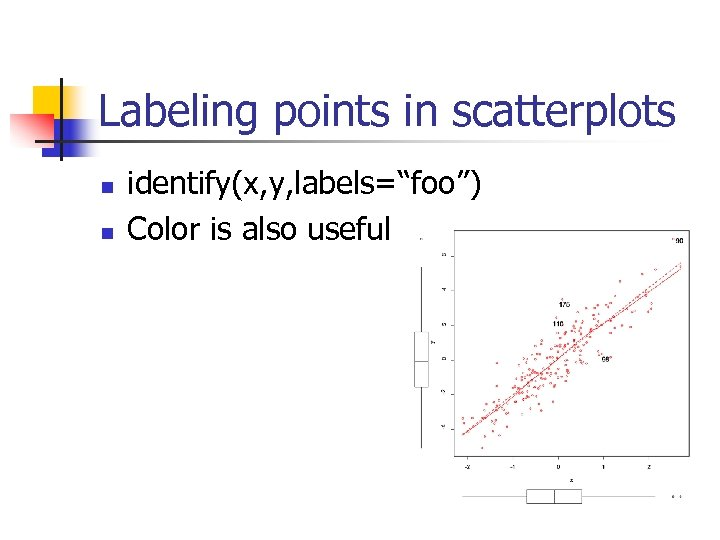Labeling points in scatterplots n n identify(x, y, labels=“foo”) Color is also useful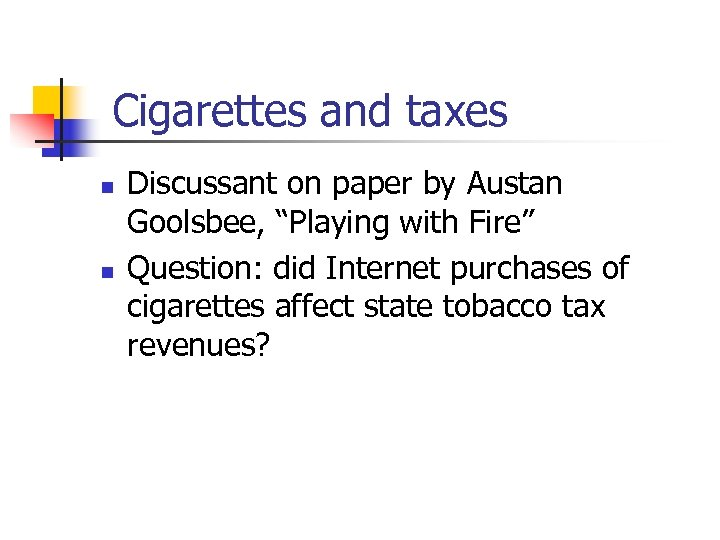Cigarettes and taxes n n Discussant on paper by Austan Goolsbee, “Playing with Fire” Question: did Internet purchases of cigarettes affect state tobacco tax revenues?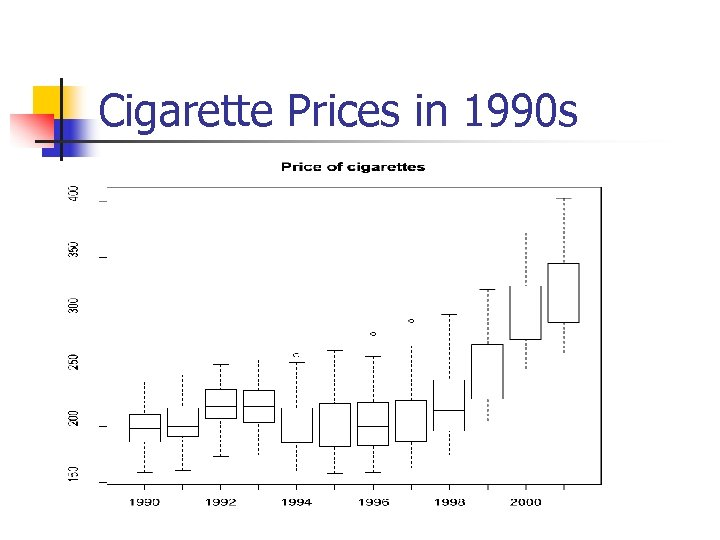Cigarette Prices in 1990 s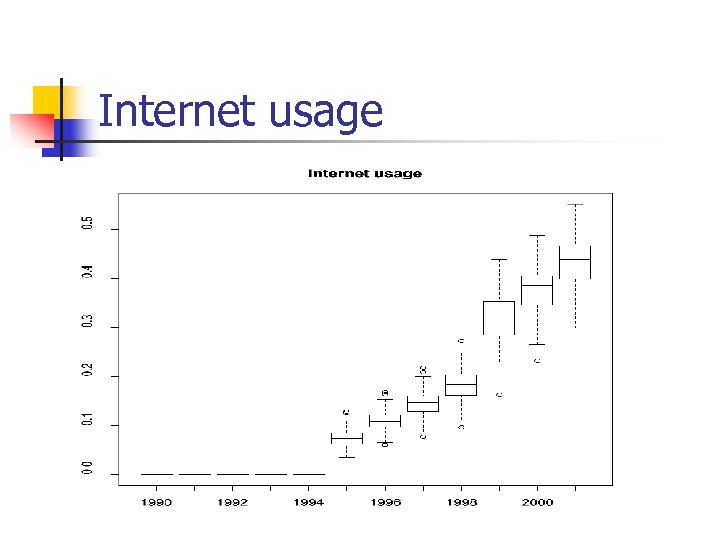Internet usage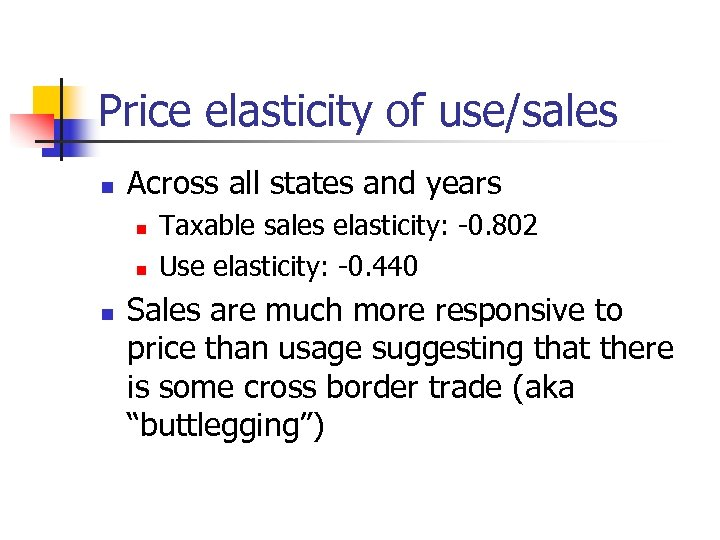Price elasticity of use/sales n Across all states and years n n n Taxable sales elasticity: -0. 802 Use elasticity: -0. 440 Sales are much more responsive to price than usage suggesting that there is some cross border trade (aka “buttlegging”)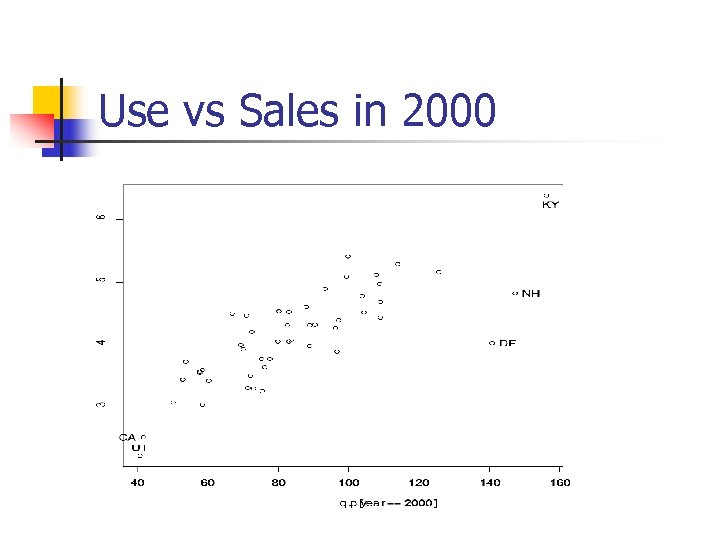Use vs Sales in 2000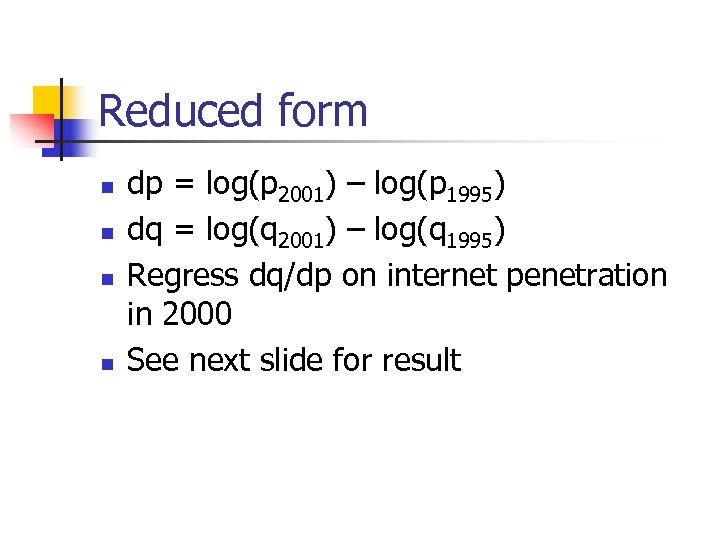Reduced form n n dp = log(p 2001) – log(p 1995) dq = log(q 2001) – log(q 1995) Regress dq/dp on internet penetration in 2000 See next slide for result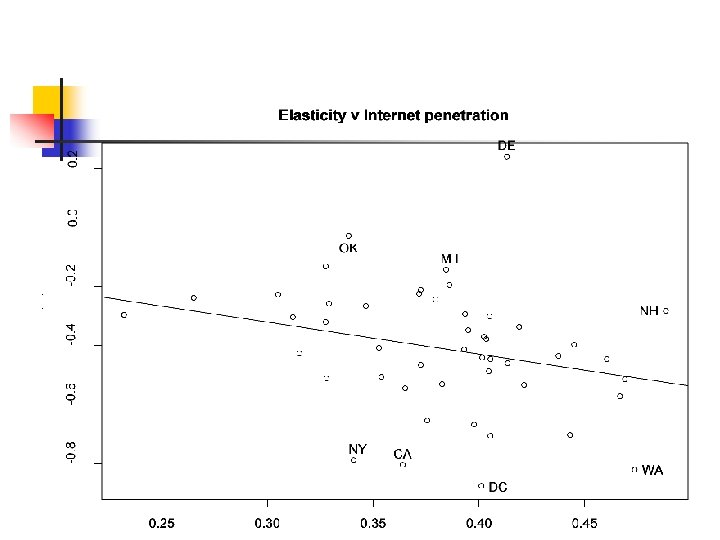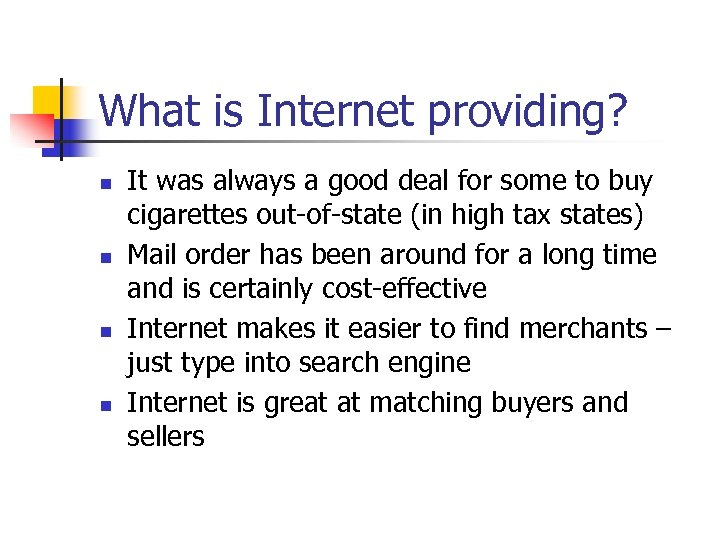What is Internet providing? n n It was always a good deal for some to buy cigarettes out-of-state (in high tax states) Mail order has been around for a long time and is certainly cost-effective Internet makes it easier to find merchants – just type into search engine Internet is great at matching buyers and sellers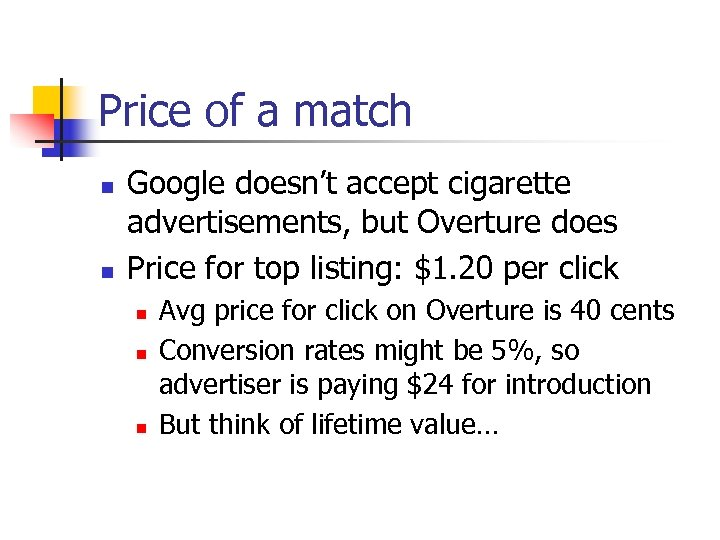Price of a match n n Google doesn’t accept cigarette advertisements, but Overture does Price for top listing: \$1. 20 per click n n n Avg price for click on Overture is 40 cents Conversion rates might be 5%, so advertiser is paying \$24 for introduction But think of lifetime value…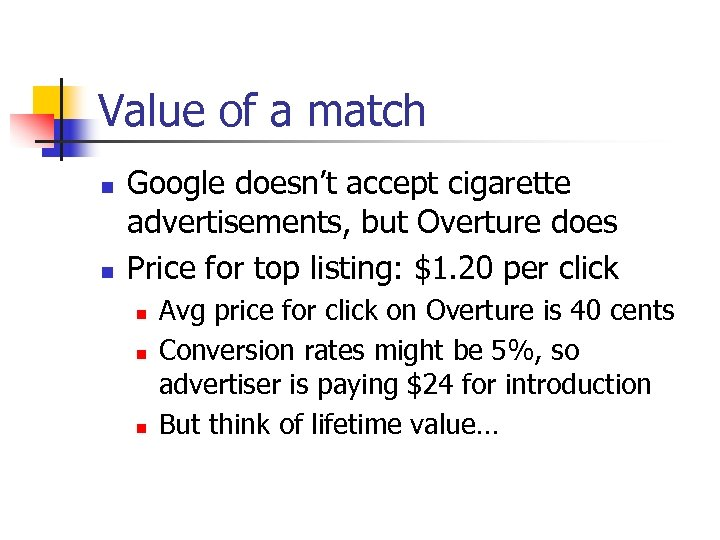Value of a match n n Google doesn’t accept cigarette advertisements, but Overture does Price for top listing: \$1. 20 per click n n n Avg price for click on Overture is 40 cents Conversion rates might be 5%, so advertiser is paying \$24 for introduction But think of lifetime value…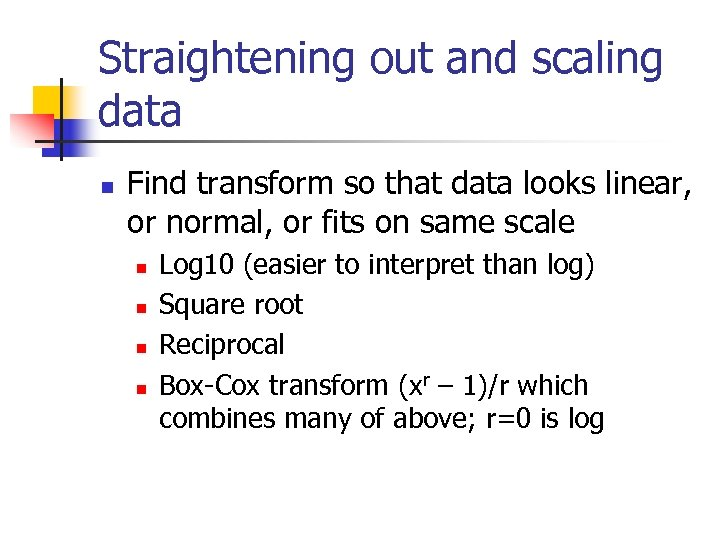Straightening out and scaling data n Find transform so that data looks linear, or normal, or fits on same scale n n Log 10 (easier to interpret than log) Square root Reciprocal Box-Cox transform (xr – 1)/r which combines many of above; r=0 is log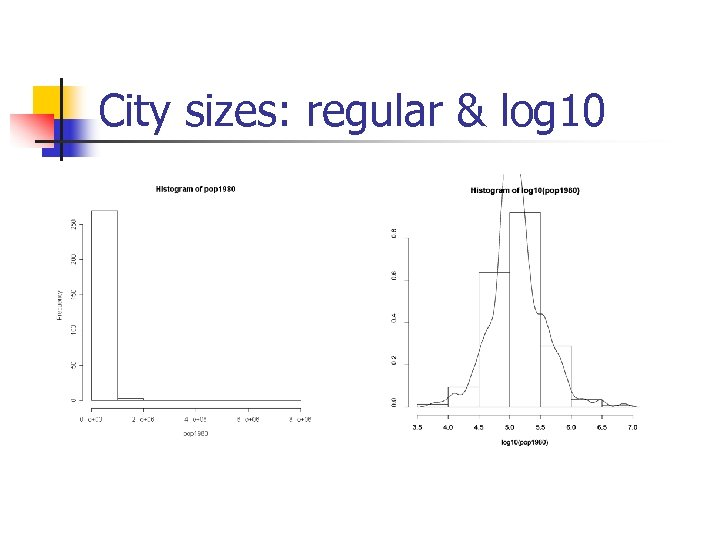City sizes: regular & log 10